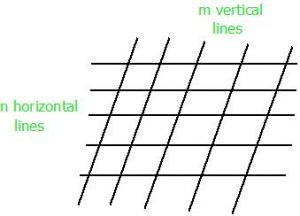GeeksforGeeks App
Open AppBrowser
Continue

# Number of parallelograms when n horizontal parallel lines intersect m vertical parallel lines

Given two positive integers n and m. The task is to count number of parallelogram that can be formed of any size when n horizontal parallel lines intersect with m vertical parallel lines.Examples:

```Input : n = 3, m = 2
Output : 3
2 parallelograms of size 1x1 and 1 parallelogram
of size 2x1.

Input : n = 5, m = 5
Output : 100```

The idea is to use Combination, which state, number of ways to choose k items from given n items is given by nCr
To form a parallelogram, we need two horizontal parallel lines and two vertical parallel lines. So, number of ways to choose two horizontal parallel lines are nC2 and number of ways to choose two vertical parallel lines are mC2. So, total number of possible parallelogram will be nC2 x mC2.
Below is C++ implementation of this approach:

## C++

 `// CPP Program to find number of parallelogram when``// n horizontal parallel lines intersect m vertical``// parallel lines.``#include``#define MAX 10``using` `namespace` `std;` `// Find value of Binomial Coefficient``int` `binomialCoeff(``int` `C[][MAX], ``int` `n, ``int` `k)``{``    ``// Calculate value of Binomial Coefficient``    ``// in bottom up manner``    ``for` `(``int` `i = 0; i <= n; i++)``    ``{``        ``for` `(``int` `j = 0; j <= min(i, k); j++)``        ``{``            ``// Base Cases``            ``if` `(j == 0 || j == i)``                ``C[i][j] = 1;`` ` `            ``// Calculate value using previously``            ``// stored values``            ``else``                ``C[i][j] = C[i-1][j-1] + C[i-1][j];``        ``}``    ``}``}` `// Return number of parallelogram when n horizontal``// parallel lines intersect m vertical parallel lines.``int` `countParallelogram(``int` `n, ``int` `m)``{``    ``int`  `C[MAX][MAX] = { 0 };   ``    ``binomialCoeff(C, max(n, m), 2);   ``    ``return` `C[n] * C[m];``}` `// Driver Program``int` `main()``{``    ``int` `n = 5, m = 5;   ``    ``cout << countParallelogram(n, m) << endl;``    ``return` `0;``}`

## Java

 `// Java Program to find number of parallelogram when``// n horizontal parallel lines intersect m vertical``// parallel lines.``class` `GFG``{``    ``static` `final` `int` `MAX = ``10``;``    ` `    ``// Find value of Binomial Coefficient``    ``static` `void` `binomialCoeff(``int` `C[][], ``int` `n, ``int` `k)``    ``{``        ``// Calculate value of Binomial Coefficient``        ``// in bottom up manner``        ``for` `(``int` `i = ``0``; i <= n; i++)``        ``{``            ``for` `(``int` `j = ``0``; j <= Math.min(i, k); j++)``            ``{``                ``// Base Cases``                ``if` `(j == ``0` `|| j == i)``                    ``C[i][j] = ``1``;``    ` `                ``// Calculate value using previously``                ``// stored values``                ``else``                    ``C[i][j] = C[i - ``1``][j - ``1``] + C[i - ``1``][j];``            ``}``        ``}``    ``}``    ` `    ``// Return number of parallelogram when n horizontal``    ``// parallel lines intersect m vertical parallel lines.``    ``static` `int` `countParallelogram(``int` `n, ``int` `m)``    ``{``        ``int` `C[][]=``new` `int``[MAX][MAX];``        ` `        ``binomialCoeff(C, Math.max(n, m), ``2``);``        ` `        ``return` `C[n][``2``] * C[m][``2``];``    ``}``    ` `    ``// Driver code``    ``public` `static` `void` `main(String arg[])``    ``{``        ``int` `n = ``5``, m = ``5``;``        ``System.out.println(countParallelogram(n, m));``    ``}``}` `// This code is contributed By Anant Agarwal.`

## Python3

 `# Python Program to find number of parallelogram when``# n horizontal parallel lines intersect m vertical``# parallel lines.``MAX` `=` `10``;` `# Find value of Binomial Coefficient``def` `binomialCoeff(C, n, k):``    ` `    ``# Calculate value of Binomial Coefficient``    ``# in bottom up manner``    ``for` `i ``in` `range``(n ``+` `1``):``        ``for` `j ``in` `range``(``0``, ``min``(i, k) ``+` `1``):``        ` `            ``# Base Cases``            ``if` `(j ``=``=` `0` `or` `j ``=``=` `i):``                ``C[i][j] ``=` `1``;` `            ``# Calculate value using previously``            ``# stored values``            ``else``:``                ``C[i][j] ``=` `C[i ``-` `1``][j ``-` `1``] ``+` `C[i ``-` `1``][j];` `# Return number of parallelogram when n horizontal``# parallel lines intersect m vertical parallel lines.``def` `countParallelogram(n, m):``    ``C ``=` `[[``0` `for` `i ``in` `range``(``MAX``)] ``for` `j ``in` `range``(``MAX``)]` `    ``binomialCoeff(C, ``max``(n, m), ``2``);` `    ``return` `C[n][``2``] ``*` `C[m][``2``];` `# Driver code``if` `__name__ ``=``=` `'__main__'``:``    ``n ``=` `5``;``    ``m ``=` `5``;``    ``print``(countParallelogram(n, m));` `# This code is contributed by 29AjayKumar`

## C#

 `// C# Program to find number of parallelogram when``// n horizontal parallel lines intersect m vertical``// parallel lines.``using` `System;` `class` `GFG``{``    ``static` `int` `MAX = 10;``    ` `    ``// Find value of Binomial Coefficient``    ``static` `void` `binomialCoeff(``int` `[,]C, ``int` `n, ``int` `k)``    ``{``        ``// Calculate value of Binomial Coefficient``        ``// in bottom up manner``        ``for` `(``int` `i = 0; i <= n; i++)``        ``{``            ``for` `(``int` `j = 0; j <= Math.Min(i, k); j++)``            ``{``                ``// Base Cases``                ``if` `(j == 0 || j == i)``                    ``C[i, j] = 1;``    ` `                ``// Calculate value using previously``                ``// stored values``                ``else``                    ``C[i, j] = C[i - 1, j - 1] + C[i - 1, j];``            ``}``        ``}``    ``}``    ` `    ``// Return number of parallelogram when n horizontal``    ``// parallel lines intersect m vertical parallel lines.``    ``static` `int` `countParallelogram(``int` `n, ``int` `m)``    ``{``        ``int` `[,]C = ``new` `int``[MAX, MAX];``        ` `        ``binomialCoeff(C, Math.Max(n, m), 2);``        ` `        ``return` `C[n, 2] * C[m, 2];``    ``}``    ` `    ``// Driver code``    ``public` `static` `void` `Main()``    ``{``        ``int` `n = 5, m = 5;``        ``Console.WriteLine(countParallelogram(n, m));``    ``}``}` `// This code is contributed By vt_m.`

## Javascript

 ``

Output:

`100`

Time Complexity: O(n2
Auxiliary Space: O(n2)
Please suggest if someone has a better solution which is more efficient in terms of space and time.